#### Quick Contact

Phone: +49 (0)8193-93700-0
info@gigahertz-optik.de

Gigahertz-Optik, Inc. (US office)
Phone: +1-978-462-1818
info-us@gigahertz-optik.com

#### Watchlist

You can add products to the watchlist and compare them with one another or send us an inquiry. There are watchlist symbols on product pages and product tables for this purpose.

# 1.10 Colorimetry

The basic problem of colorimetry is the quantification of the physiological color perception caused by a certain spectral color stimulus function φλ(λ). When the color of a primary light source has to be characterized, φλ(λ) equals the source’s spectral radiant power Φλ(λ) (or another spectral radiometric quantity, such as radiant intensity or radiance). When the color of a reflecting or transmitting object (for example a filter) has to be characterized, φλ(λ) equals the incident spectral irradiance impinging upon the object’s surface, multiplied by the object’s spectral reflectance, its spectral radiance coefficient or its spectral transmittance. Since colors of reflecting or transmitting objects depend on the object’s illumination, the CIE has defined colorimetric standard illuminants. The CIE Standard Illuminant A is defined by a Planckian blackbody radiator at a temperature of 2856 K, and the CIE Standard Illuminant D56 is representative of average daylight with a correlated color temperature of 6500 K (for the definition of color temperature, see below).

### RGB and XYZ color matching functions

According to the tristimulus theory, every color which can be perceived by the normal-sighted human eye can be described by three numbers that quantify the stimulation of red, green and blue cones. If two color stimuli result in the same values for these three numbers, they produce the same color perception even when their spectral distributions are different. Around 1930, Wright and Guild performed experiments during which observers had to combine light at 435.8 nm, 546.1 nm and 700 nm in such a way that the resulting color perception matched the color perception produced by monochromatic light at a certain wavelength of the visible spectrum. Evaluation of these experiments resulted in the definition of the standardized RGB color matching functions r(λ),  g (λ) and b(λ), which have been transformed into the CIE 1931 XYZ color matching functions x(λ), y (λ) and z(λ). These color matching functions define the CIE 1931 standard colorimetric observer and are valid for an observer’s field of view of 2°. Practically, this observer can be used for any field of view smaller than 4°. For a 10 ° field of view, the CIE specifies another set of color matching functions x10(λ), y10(λ) and z(λ)10. This set defines the CIE 1964 supplementary standard colorimetric observer, which has to be used for fields of view larger than 4 °.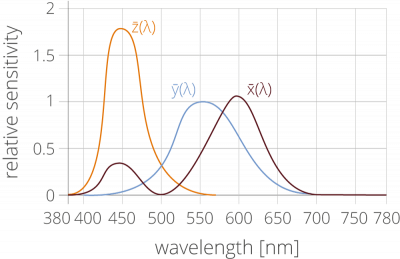Fig. 1: XYZ color matching functions as defined by the CIE 1931 standard colorimetric observer.
x(λ) (solid black line) consists of a short- and a longwavelength part, and
y(λ) (solid grey line) is identical with the CIE spectral luminous efficiency function V(λ).

Although RGB and XYZ color matching functions can be equally used to define three parameters where the numbers uniquely describe a certain color perception. The XYZ color matching functions are preferred because they have positive values for all wavelengths (Fig. 1). In addition, y (λ) is equal to the CIE spectral luminous efficiency function V(λ) for photopic vision.

The XYZ tristimulus values of a certain spectral color stimulus function φλ (λ)  are calculated by

 X = k ∫ φλ(λ) × x (λ) dλ λ
 Y = k ∫ φλ(λ) ×  y (λ) dλ λ
 Z = k ∫ φλ(λ) ×  z (λ) dλ λ

The choice of the normalization constant k depends on the colorimetric task: When the spectral color stimulus φλ (λ)  describes a spectral radiometric quantity of a primary light source, k = 683 lm/W and consequently Y yields the corresponding photometric quantity. When the spectral color stimulus  φλ (λ)  describes the spectral distribution of optical radiation reflected or transmitted by an object, k is defined by

 k = 100 ∫ Eλ(λ) y (λ) dλ λ

with E(λ) denoting the incident spectral irradiance impinging upon the object’s surface.

### The (x, y) and (u’, v’) chromaticity diagrams

Although the XYZ tristimulus values define a three-dimensional color space representing all possible color perceptions, the representation of color in a two-dimensional plane is often sufficient for most applications. One possibility for a twodimensional representation is the CIE 1931 (x, y) chromaticity diagram with its coordinates x and y calculated from a projection of the X, Y and Z values:

 x = X X + Y + Z
 y = Y X + Y + Z

Although widely used, the (x, y) chromaticity diagram is largely limited by non-uniformity since geometric distances in the (x, y) chromaticity diagram do not correspond to perceived color differences. It is for this reason that in 1976, the CIE defined the uniform (u’, v’) chromaticity scale (UCS) diagram, with its coordinates defined by

 u' = 4X X + 15Y + 3Z
 v' = 9Y X + 15Y + 3Z

Although this definition of the u’ and v’ coordinates does not provide a strict correspondence between geometric distances and perceived color differences, there are far less discrepancies than in the CIE (x, y) chromaticity diagram.Fig. 2: The CIE 1931 (x,y) chromaticity diagram

Source (valid as of 2002): http://home.wanadoo.nl/paulschils/10.02.htm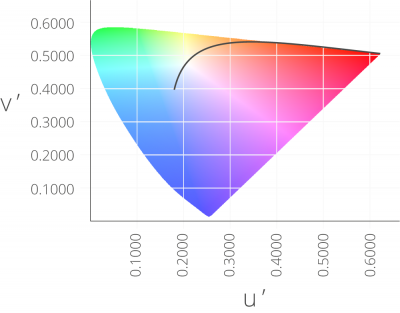Fig. 3: The CIE 1976 (u’, v’) chromaticity diagram.

Source (valid as of 2002): http://home.wanadoo.nl/paulschils/10.02.htm

### Correlated color temperature

The correlated color temperature is used to characterize the spectral distribution of optical radiation emitted by a light source. This characterization corresponds to the projection of a two-dimensional chromaticity diagram onto a onedimensional scale and is therefore very coarse.

In detail, the correlated color temperature is given in Kelvin (K) and is the temperature of the blackbody (Planckian) radiator whose received color most closely resembles that of a given color stimulus.

As a (simplified) rule of thumb, spectral distributions dominated by long (reddish) wavelengths correspond to a low correlated color temperature whereas spectral distributions dominated by short (bluish) wavelengths correspond to a high correlated color temperature. For example, the warm color of incandescent lamps has a correlated color temperature of about 2800 K, average daylight has a correlated color temperature of about 6500 K and the bluish white from a Cathode Ray Tube (CRT) has a correlated color temperature of about 9000 K.

### Color rendering index CRI

The color rendering index (CRI) is a numerical description of the color rendition quality of a light source at the identical correlated color temperature. Here, the general color rendering index Ra represents the average of the first eight test color samples. Overall, 14 such test color samples that were defined by DIN 6169 and CIE 13.2. are currently used. In many cases, an  additional CRI 15 that was added subsequently is also calculated. In order to perform the calculation, a black body radiator’s color temperature of up to 5000 K is used. For temperatures above 5000K, daylight e.g. D65 (D65 = 6500 K daylight) is used instead. Fundamentally, the color rendering index does not depend on the color temperature. It only depends on the relation the light source’s spectral distribution within the visible spectral range to that of a reference light source. Mathematically speaking Ra is defined by:

Ra = 1/8 Σ8i=1 Ri

where Ri are given by

Ri = 100 - 4,6 ×  Δ Ei

Here, ΔEi is the Euclidian distance of the respective test color sample when illuminated by the light source under test as compared to the reference light source. Fig. 4 shows the spectral functions of the test color samples.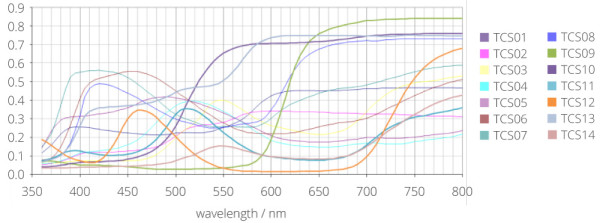Fig. 4: Test color samples according to CIE 13.2

### Color preference and rendition metric CQS

In addition to the CRI specified by CIE 1976, there are 15 test color samples of the colorquality scale (CQS) that are calculated in a similar way. The CQS method (version 7.5) uses 15 selected (saturated) test colors from the Munsell color system instead of the CIE test color samples. According to the CIE color rendering index, test light sources that increase the saturation of an object’s hue as compared to a reference light source are evaluated with low CRI scores. Unlike the CQS reference light source, the CQS method does not evaluate an increase in saturation of a test device in a negative way in order to take into account an observer’s tendency to prefer colors with a higher saturation. An increased saturation of the color rendition when an object is illuminated by a light source under test means a change in color perception. This again means that there is no conformity of the test light source and the reference thus leading to a decreased CRI score. By definition, the color quality scale is hence no pure color rendition metric. Instead, it is a combination of color preference and rendition metric. Its advantage is that the color preference is taken into account. At the same time, an objective evaluation of color rendition is not possible.

### Planckian locus

The Planckian locus is a diagrammatic representation of a black body radiator’s emission with respect to the color temperature (see Fig. 5).Fig. 5: Planckian black body locus

### The dominant wavelength of a radiator

The dominant wavelength of a radiator is determined by the point where a straight line from the white point through the color coordinates of the radiator intersects with the spectral locus, the outer curved boundary of the CIE 1931 color space diagram. This is shown in Figure 6. The dominant wavelength cannot be determined directly from the spectrum. Instead, it represents an evaluation of a light source’s properties based on the color metric.Fig. 6: Dominant wavelength in the CIE 1931 color space diagram

### The purity of a radiator

The purity defines how close the color coordinate of a radiator is positioned in relation to the spectral locus in the CIE color space diagram. Geometrically, it can be depicted as shown in Figure 7.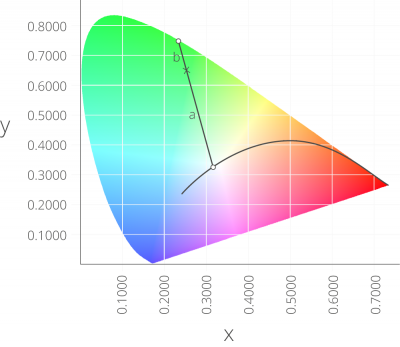Fig. 7: Purity in CIE 1931 color space diagram

The color coordinate of the measurement is represented by the cross symbol. A line is drawn from the white point via the color coordinate towards the dominant wavelength of the measurement. The purity is now calculated as follows by evaluation of the sections of the line:

 purity = a a + b'

where a is the distance between the white point and the measurement point and b is the distance between the measurement point and the dominant wavelength. If the purity equals one, i. e. b equals zero, the light source has a pure single line spectrum e. g. a laser. If purity equals zero the radiator has a spectrum that is as broad as possible.

Distances in the xy color space, which was defined in 1931 by CIE, do not reflect the distances as perceived by the human eye. This means that if two measurement points in the color diagram have the same distance to an arbitrary reference point, the perceived color contrast differs in general. In 1942, MacAdam tried to take this into account by adding ellipses into the color diagram as shown in Figure 8. Nowadays, so-called n step MacAdam ellipses are used. Here, n is the magnification of the ellipse in comparison to the one which was originally defined by MacAdam. Established values are 3x, 5x and 10x.Fig. 8: MacAdam ellipses in xy color space

MacAdam’s research presented a huge progress and considering the options at that time in terms of experimental setups and computing power, his results are truly remarkable. In the 1960s, additional research was promoted which resulted in the CIE 1976 u'v' color space. Although the xy color space is the most widely accepted color space to date, CIE recommends using the u'v' color space.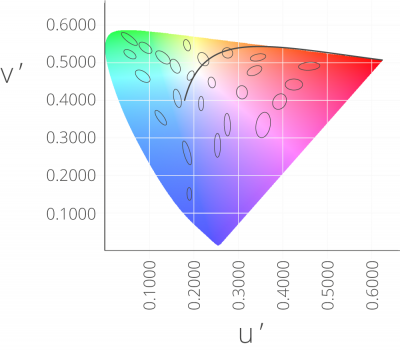Fig. 9: MacAdam ellipses in u’v’ color space

Recent findings indicate that the ellipses are not the ideal choice for modern solid state lighting (SSL) luminaire technology such as light emitting diodes (LEDs). The original ellipses were determined using fluorescent lamps of six different color temperatures. Recent technologies are not subjected to the same restrictions. Thus, new regulations are required example e.g. as defined by ANSI (ANSI NEMA ANSLG. 2011. C78.377-2008) using eight nominal CCTs as well as CCTs in 100K steps (see Fig. 9).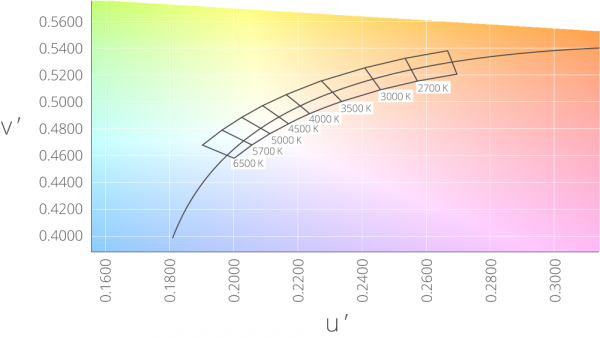Fig. 10: ANSI bins in the u’v’ diagram

Most recent recommendations by the CIE (IEC 60081, IEC 1997) include the use of n step circles in u'v' color space with their centers at the position of the MacAdam ellipses. The radius of these circles can be adjusted as required. Their mathematical description is given by:

( u' - u'c )2 + ( v' - v'c )2 = (0.0011 × n)2

where u'C and v'C are their centre coordinates. With such a description, interpolation in terms of CCT becomes an option which reflects the diversity of LED technology. For binning purposes, either these or customized areas defined by the manufacturers are used in the selection and sorting of LEDs based on specific color properties. Such measurements require precise spectrometers that have a high wavelength precision, narrow optical bandwidth (or a bandwidth correction) and a verified absolute calibration. Additionally, highly sensitive devices that enable rapid measurements and fast data transfers are required. For a measurement device to be used in LED binning, it must meet these speed and precision requirements. Figure 11 shows the color bins of a LED manufacturer in the u'v' color space.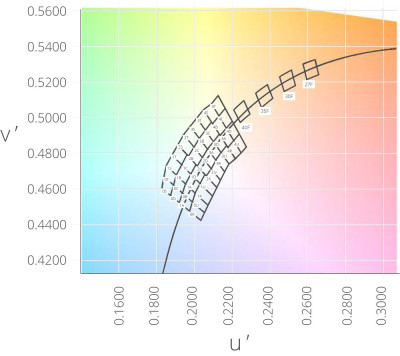Fig. 11: Color bins of a LED manufacturer